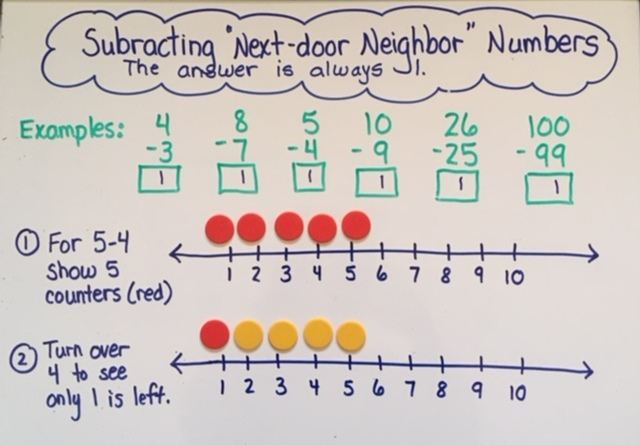# Ten Frames Part 2: Addition and subtraction

Last week’s focus was on using ten frames to help with students’ number sense and conceptual development of number bonds for amounts 1-10.This post will feature ways to use ten frames to enhance students’ understanding of addition and subtraction. Look for freebies and a video!

There are many addition and subtraction strategies to help students memorize the basic facts such as these below. The ten frame is a very good tool for students of all grade levels to make these strategies more concrete and visual. I will focus on some of these today.

• add or take away 1 (or 2)
• doubles, near doubles
• facts of 10
• make a ten
• add or sub. tens and ones

Doubles and near doubles (doubles +1, -1, +2, or -2): If the doubles are memorized, then problems near doubles can be solved strategically.

• Show a doubles fact on a single ten frame (for up to 5 + 5).  Use a double ten-frame template for 6 + 6 and beyond.
• With the same doubles fact showing, show a near doubles problem.  This should help students see that the answer is just one or two more or less.
• Repeat with other examples.
• Help student identify what a doubles + 1 more (or less) problem looks like. They often have a misconception there should be a 1 in the problem. Make sure they can explain where the “1” does come from. Examples:  7 + 8, 10+11, 24+25, 15 +16, etc.
• For subtraction, start with the doubles problem showing and turn over the 2-color counters or remove them.

Facts of 10: These are important to grasp for higher level addition / subtraction problems as well as rounding concepts. Continue reading

# Addition and Subtraction Part 3: Facts Strategies KG-3rd

by C. Elkins, OK Math and Reading LadyThis is part three in a series of strategies regarding addition and subtraction strategies.  This part will focus on a variety of strategies to help toward memorization of facts, meaning automatic computation. While children are learning their number bonds (building up to 5 in KG, to 10 in first grade, and to 20 in second grade), there are other facts which cross several number bonds that students can work towards. These strategies to build mental math automaticity are highlighted below. Get some freebies in the section on doubles / near doubles.

Identity (or Zero) Property:

• The value of the number does not change when zero is added or subtracted.
• 3 + 0 = 3
• 9 – 0 = 9

Subtracting All:

• The answer is always zero when you take away / subtract all.
• 9 – 9 = 0
• 50 – 50 = 0

• Adding 1 results in the next number in the counting sequence.
• Subtracting 1 means naming the number that comes right before it in the counting sequence.
• With manipulatives, lay out an amount for student to count.  Slide one more and see if he/she can name the amount without recounting.
• Do the same as above, but take one away from the group to see if he/she can name the amount without recounting.
• Show this concept using a number line.
• 6 + 1 = 7;    26 + 1 = 27
• 7 – 1 = 6;     37 – 1 = 36
• After +1 or -1 strategies are in place, then go for +2 or -2 for automatic processing.

Next-Door Neighbor Numbers:

• If subtracting two sequential numbers (ie 7 subtract 6), the answer is always one because you are taking away almost all of the original amount.
• Help students identify these types of problems:  8-7;   10-9;   98-97;  158-157
• Guide students to writing these types of problems.
• Relate these to subtracting 1 problems.  If 10-1 = 9;   then 10 – 9 = 1.
• Show on a number line.